# Split field on first number

3062
11
01-14-2016 02:32 PMOccasional Contributor

Edit (by Xander Bakker): the original question was placed at this thread: Extracting an integer from a string field but I decided to branch it to a new thread.

I have a similar situation...

For Example:

s ="POWERS 1C-23HZ"

and some are like s2 = RATTLER4C-34HZ

I would like to tell python to find the first number in each field and then split the field at the same location at the first number. so....

Field 1 = RATTLER

Field 2 = 4C-34HZ

derived from the string above: s2

I would like to use the field calculator to do this.

I know you can use this to split on the space:

`!field! = s.split(' ')`

I did this in the field calculator expression box and it works but only on the strings with a space where I need to break it.

Any help is much appreciated!

Dan A.

Message was edited by: Xander Bakker. Post branched to new Thread

Tags (3)
1 Solution

Accepted SolutionsbyEsri Esteemed Contributor

A small addition to the code contributed by Darren. In the example  "POWERS 1C-23HZ", your first result will include "POWERS " with the space included. If any space at the beginning or end should be stripped, you can use something like this

```a = "POWERS 1C-23HZ"
for i in range(len(a)):
if a.isdigit():
b = [a.strip() for a in [a[:i],a]
break
print b

#  ['POWERS ', '1C-23HZ']```

If you want to use this in the field calculator and fill two fields with it, you must remember that a Field Calculation can only write to a single field per run. Does than mean that you need two functions to implement  what you want? No, not really. You can work with an additional parameter (index) that will extract the value you want.

The code block could be something like:

```def SplitAtDigit(a, index):
try:
found = False
for i in range(len(a)):
if a.isdigit():
b = [a.strip() for a in [a[:i], a]
found = True
break
if found:
return b[index]
else:
if index==0:
return a
else:
return ''
except:
return ''```

and you can use these settings: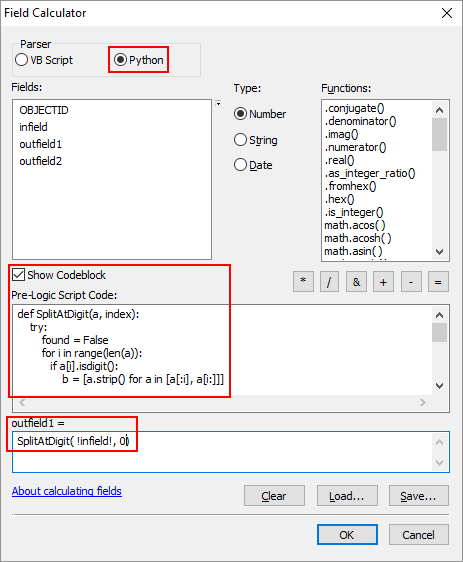for the first field and:

`SplitAtDigit( !infield!, 1)`

...for the second field. This will result in: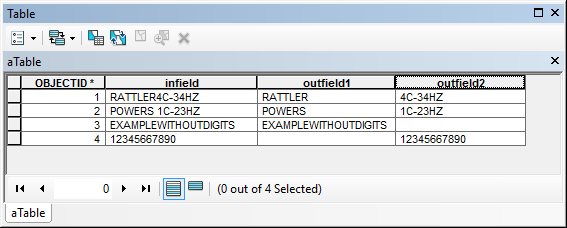11 RepliesbyMVP Legendary Contributor

>>> a = "RATTLER4C-34HZ"

>>> b=a.split("-")[-2]

>>> b

'4'byMVP Honored Contributor
```>>> a = "RATTLER4C-34HZ"
... for i in range(len(a)):
...    if a.isdigit():
...        b = [a[:i],a
...        break
... print b
...
['RATTLER', '4C-34HZ']```byEsri Esteemed Contributor

A small addition to the code contributed by Darren. In the example  "POWERS 1C-23HZ", your first result will include "POWERS " with the space included. If any space at the beginning or end should be stripped, you can use something like this

```a = "POWERS 1C-23HZ"
for i in range(len(a)):
if a.isdigit():
b = [a.strip() for a in [a[:i],a]
break
print b

#  ['POWERS ', '1C-23HZ']```

If you want to use this in the field calculator and fill two fields with it, you must remember that a Field Calculation can only write to a single field per run. Does than mean that you need two functions to implement  what you want? No, not really. You can work with an additional parameter (index) that will extract the value you want.

The code block could be something like:

```def SplitAtDigit(a, index):
try:
found = False
for i in range(len(a)):
if a.isdigit():
b = [a.strip() for a in [a[:i], a]
found = True
break
if found:
return b[index]
else:
if index==0:
return a
else:
return ''
except:
return ''```

and you can use these settings:for the first field and:

`SplitAtDigit( !infield!, 1)`

...for the second field. This will result in:byMVP Legendary Contributor

Xander, good thing you branched it, poor Phil is probably wondering about the emails he is going to get tomorrow morning on a 3 year old threadOccasional Contributor

Xander et al...

This is the code i entered into the code block:

```def SplitAtDigit(a,index):
try:
found = False
for i in range(len(a)):
if a.isdigit():
b = [a.strip() for a in [a[:i], a]
found = True
break
if found:
return b[index]
else:
if index ==0:
return a
else:
return ' '
except:
return ' '```

and then:

`SplitAtDigit( !field!,0)`

unfortunately all it does is copy one field into the new field without splitting the original text string.

i have compared this with what you posted many times and it took me several tries to get the indentation right. i know from the table you posted it worked for you, so I son't understand why it isn't working for me.

All fields i'm using are text fields and i don't see anything else that would keep this from working.

the only other problem i can think of is that some of strings in the original text column have multiple spaces before the first number.

Let me know if there is anything jumping out at you in this block of code.

DanbyEsri Esteemed Contributor

Can you post a sample of your data? What is the error you obtain?

You should't have any problems with the indentation, but I can create an expression file that you can load.byEsri Esteemed Contributor

Find attached the Expression file. In the Field Calculator, click on load: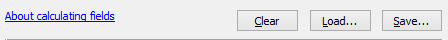Make sue you switch to the Python parser: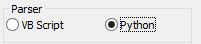In the part below change the index (0 or 1) according to the field you want to fill: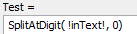Occasional Contributor

Xander,

that worked perfectly!

All except where the text begins with a number such as:

70 RANCH USX BB 09-99HZ

3D 16N-21HZ

the script copied the entire string into the field that used the "1" selection...which makes perfect sense. since it found the first number and printed everything past it.

There are only a few records that start with a number so i can fix it easily.

The difference between mine and the calc file you sent is you had a single " at the end of the final returns. I had used ' '

I'm assuming there's a way to tell the script to split it at the first second or third number....by adding a ...etc... somewhere in line 6?

Now it's more of trying to understand how the script actually works.byMVP Legendary Contributor

Darren is right, his is probably easier to understand...  but for continuity

```>>> a = "RATTLER4C-34HZ"
>>> b = a.split("-")[-2]
>>> b
'4'
>>> c="4"+a.split("4",1)
>>> c
'4C-34HZ'```

The number after the "4" is the maximum number of times to split the string, incase you have more than one four, which you do